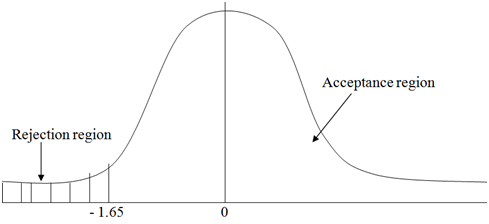## Standard hypothesis tests, Mathematics

Assignment Help:

Standard Hypothesis Tests

In principal, we can test the significance of any statistic related to any type of probability distribution. Conversely we will be interested in a few standard cases. The sample statistics mean, proportion and variance, are concerned the normal, F, t, and chi-squared distributions

Hence

1.  Normal test

Test a sample mean (x¯) against a population mean (µ) whereas samples size n > 30 and population variance σ2 is identified and sample proportion, P whereas sample size np >5 and nq >5 because in this case the normal distribution can be utilized to approximate the binomial distribution

2.   t test

Tests a sample mean (x¯) against a population mean and especially where the population variance is un-known and also n < 30.

3.   Variance ratio test or f test

This is used to compare population variances and it is used along with samples of any size drawn from normal populations.

4.  Chi squared test

This can be used to test the association among attributes or the goodness of fit of an observed frequency distribution to a standard distribution

Illustration 1

A specific NGO carried out a survey in a specific community in order to establish the average at which the girls are married. The results of the survey specified that the marriage age for the girls is 19 years

In order to establish the validity of the mean marital age, a sample of 50 women was interviewed and the average age specified that they got married at the age of 16 years. Conversely the different ages at which they were married differed along with the standard deviation of 2.1years

The sample data specified that the marital age is less 19 years. Is this conclusion true or not true?

Required

Conduct a statistical test to either support the above conclusion drawn from the sample statistics that is the marriage age is less than 19 years, use a level of significance of 5 percent

Solution

1.   Null hypothesis

H0: μ (mean marital age) = 19 years

Alternative hypothesis      H1: μ (mean marital age) < 19 years

2.   The level of significance is 5 percent

3.   The test statistics is the sample mean age, x¯ = 16 years

4.   The critical value of the one tailed test as one tailed as the alternative hypothesis is an inequality at 5 percent level of significance is -1.651.      The standardizes value of the sample mean is

Z =  (x¯ - μ)/ S

Whereas S = s/√n

Whereas, x¯ = Sample mean

µ = Population mean

S = sample standard deviation

n = sample size

z = standard value as per computation

The standard value Z must fall in the acceptance region for us to accept the null hypothesis.  Hence it must be > - 1.65 otherwise we accept the alternative hypothesis.

Z = (16-19)/(2.1/√50) = - 10.1

2.      As -10.1 < -1.65, we reject the null hypothesis but accept the alternative hypothesis at 5 percent level of significance that is the marriage age in this community is significantly lower than 19 years

#### Capture a curvature in the relationship - quadratic model, 1. Consider the ...

1. Consider the model Y t = β 0 + β 1 X t + ε t , where t = 1,..., n.  If the errors ε t are not correlated, then the OLS estimates of  β 0   and β

#### Pythagorean theorem, How do you find the perimeter of an irregular shape us...

How do you find the perimeter of an irregular shape using Pythagorean theorem?

#### Square and square root., the value of square root of 200multiplied by squar...

the value of square root of 200multiplied by square root of 5+

#### Example of parametric equations and parametric curves, Draw the parametric ...

Draw the parametric curve for the subsequent set of parametric equations. X = t 2 +t Y=2t-1 -1 t 1 Solution Note that the only dissimilarity here is the exis

#### Calculus with matrices, Calculus with Matrices There actually isn't a ...

Calculus with Matrices There actually isn't a whole lot to it other than to just ensure that we can deal along with calculus with matrices. Firstly, to this point we've onl

#### External forces, It is the catch all force. If there are some other forces ...

It is the catch all force. If there are some other forces which we decide we need to act on our object we lump them in now and call this good. We classically call F(t) the forcing

#### Give an examples of simplifying fractions , Give an examples of Simplifying...

Give an examples of Simplifying Fractions ? When a fraction cannot be reduced any further, the fraction is in its simplest form. To reduce a fraction to its simplest form,

#### Houses having the floor , Suppose you are in the market for a new home and ...

Suppose you are in the market for a new home and are interested in a new housing community under construction in a another city. a) The sales representative informs you that the

98+3

#### Shares nd dividends, conclusion for the shares nd dividends

conclusion for the shares nd dividends